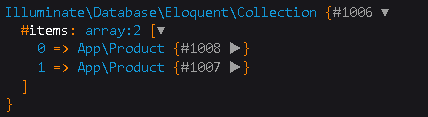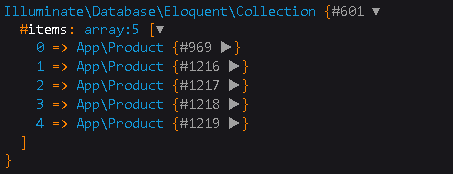# Exploring Laravel Eloquent: whereIn & whereNotIn - Let's Begin!

0

## How to use whereIn and whereNotIn Query in LaravelIn this article we will learn how can you use whereIn and whereNotIn query in Laravel . We will learn the multiple way to use whereIn and whereNotIn query and how it is different from simple SQL Query .

whereIn method basically takes two parameter one is the ' column_name ' and the other one is an array having choices . Laravel finds throughout the table and return the rows whose column values matches with the values given inside the array .

whereNotIn method is also takes two parameter similar to whereIn and returns the rows having column values that are not matched with the array values .

For better understanding i will take an example of a product table which is shown below.

### Laravel Eloquent whereIn :

#### SQL Query :

`SELECT * FROM products`
```
WHERE price IN ( 99,10,78 );
```

#### Laravel Syntax 1:

```\$data=Model_Name::select('*')

->whereIn('column_name',[ value1,value2,value3 ])

->get();

dd(\$data);
```

#### Laravel Example 1:

```\$data=Product::select('name')

->whereIn('price',[ 99,10,78 ])

->get();

dd(\$data);
```
```
```

#### Laravel Syntax 2:

```\$data=DB::table('table_name')

->whereIn('column_name',[ value1,value2,value3 ])

->get();

dd(\$data);
```

#### Laravel Example 2:

```\$data=DB::table('products')

->whereIn('price',[ 99,10,78 ])

->get();

dd(\$data);
```

### Output :### Laravel Eloquent whereNotIn :

#### SQL Query :

`SELECT * FROM products`
```
WHERE price NOT IN ( 99,10,78 );
```

#### Laravel Syntax 1:

```\$data=Model_Name::select('*')

->whereNotIn('column_name',[ value1,value2,value3 ])

->get();

dd(\$data);
```

#### Laravel Example 1:

```\$data=Product::select('name')

->whereNotIn('price',[ 99,10,78 ])

->get();

dd(\$data);
```
```
```

#### Laravel Syntax 2:

```\$data=DB::table('table_name')

->whereNotIn('column_name',[ value1,value2,value3 ])

->get();

dd(\$data);
```

#### Laravel Example 2:

```\$data=DB::table('products')

->whereNotIn('price',[ 99,10,78 ])

->get();

dd(\$data);
```

### Output :These are the different ways to use whereIn and whereNotIn method in Laravel . Hope this article helped you .

For any query do not hesitate to comment 💬

whereBetween and whereNotBetween in Laravel

Get average in Laravel Eloquent

Search functionality in Laravel

Laravel 7/6 Email Verification

Why Laravel is so Popular PHP Framework

Laravel 7 PDF Generation ( DomPDF )